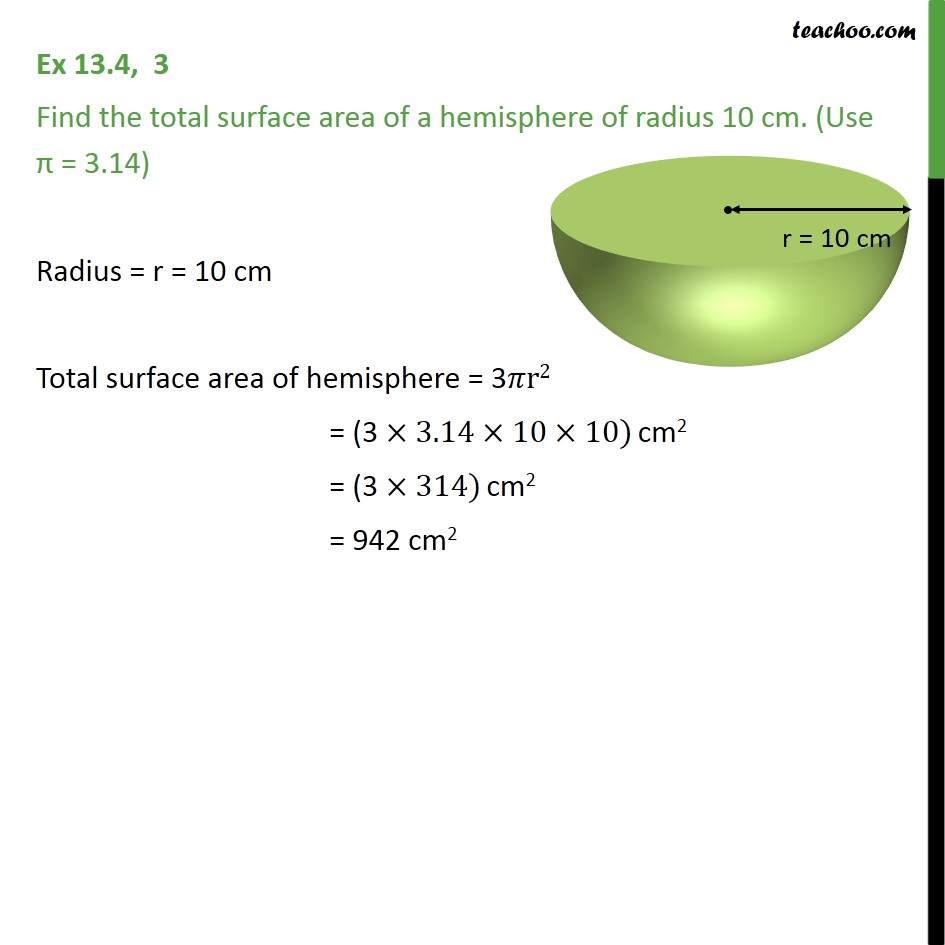Chapter 13 Class 9 Surface Areas and Volumes

Class 9
Important Questions for Exam - Class 9Learn in your speed, with individual attention - Teachoo Maths 1-on-1 Class

### Transcript

Ex 11.2, 3 Find the total surface area of a hemisphere of radius 10 cm. (Use = 3.14) Radius = r = 10 cm Total surface area of hemisphere = 3 r2 = (3 3.14 10 10) cm2 = (3 314) cm2 = 942 cm2